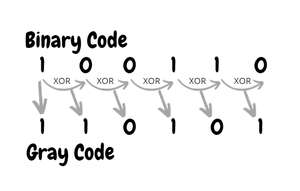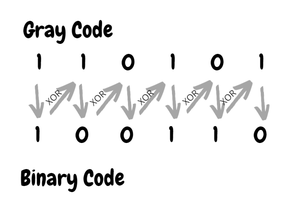GeeksforGeeks App
Open AppBrowser
Continue

# Gray to Binary and Binary to Gray conversion

Binary Number is the default way to store numbers, but in many applications, binary numbers are difficult to use and a variety of binary numbers is needed. This is where Gray codes are very useful.

Gray code has a property that two successive numbers differ in only one bit because of this property gray code does the cycling through various states with minimal effort and is used in K-maps, error correction, communication, etc.

How to generate n bit Gray Codes?

```Following is 2-bit sequence (n = 2)
00 01 11 10
Following is 3-bit sequence (n = 3)
000 001 011 010 110 111 101 100
And Following is 4-bit sequence (n = 4)
0000 0001 0011 0010 0110 0111 0101 0100 1100 1101 1111
1110 1010 1011 1001 1000```

n-bit Gray Codes can be generated from a list of (n-1)-bit Gray codes using the following steps.

1. Let the list of (n-1)-bit Gray codes be L1. Create another list L2 which is the reverse of L1.
2. Modify the list L1 by prefixing a ‘0’ in all codes of L1.
3. Modify the list L2 by prefixing a ‘1’ in all codes of L2.
4. Concatenate L1 and L2. The concatenated list is the required list of n-bit Gray codes.

Please refer Generate n-bit Gray Codes for a detailed program.

How to Convert Binary To Gray and Vice Versa?

```Binary : 0011
Gray   : 0010

Binary : 01001
Gray   : 01101```

In computer science many a time we need to convert binary code to gray code and vice versa. This conversion can be done by applying the following rules :

Binary to Gray conversion :

1. The Most Significant Bit (MSB) of the gray code is always equal to the MSB of the given binary code.
2. Other bits of the output gray code can be obtained by XORing binary code bit at that index and previous index.Binary code to gray code conversion

Gray to binary conversion :

1. The Most Significant Bit (MSB) of the binary code is always equal to the MSB of the given gray code.
2. Other bits of the output binary code can be obtained by checking the gray code bit at that index. If the current gray code bit is 0, then copy the previous binary code bit, else copy the invert of the previous binary code bit.Gray code to binary code conversion

Below is the implementation of the above steps.

## C++

 `// C++ program for Binary To Gray``// and Gray to Binary conversion``#include ``using` `namespace` `std;` `// Helper function to xor two characters``char` `xor_c(``char` `a, ``char` `b) { ``return` `(a == b) ? ``'0'` `: ``'1'``; }` `// Helper function to flip the bit``char` `flip(``char` `c) { ``return` `(c == ``'0'``) ? ``'1'` `: ``'0'``; }` `// function to convert binary string``// to gray string``string binarytoGray(string binary)``{``    ``string gray = ``""``;` `    ``// MSB of gray code is same as binary code``    ``gray += binary;` `    ``// Compute remaining bits, next bit is computed by``    ``// doing XOR of previous and current in Binary``    ``for` `(``int` `i = 1; i < binary.length(); i++) {``        ``// Concatenate XOR of previous bit``        ``// with current bit``        ``gray += xor_c(binary[i - 1], binary[i]);``    ``}` `    ``return` `gray;``}` `// function to convert gray code string``// to binary string``string graytoBinary(string gray)``{``    ``string binary = ``""``;` `    ``// MSB of binary code is same as gray code``    ``binary += gray;` `    ``// Compute remaining bits``    ``for` `(``int` `i = 1; i < gray.length(); i++) {``        ``// If current bit is 0, concatenate``        ``// previous bit``        ``if` `(gray[i] == ``'0'``)``            ``binary += binary[i - 1];` `        ``// Else, concatenate invert of``        ``// previous bit``        ``else``            ``binary += flip(binary[i - 1]);``    ``}` `    ``return` `binary;``}` `// Driver program to test above functions``int` `main()``{``    ``string binary = ``"01001"``;``    ``cout << ``"Gray code of "` `<< binary << ``" is "``         ``<< binarytoGray(binary) << endl;` `    ``string gray = ``"01101"``;``    ``cout << ``"Binary code of "` `<< gray << ``" is "``         ``<< graytoBinary(gray) << endl;``    ``return` `0;``}`

## Java

 `// Java program for Binary To Gray``// and Gray to Binary conversion``import` `java.io.*;``class` `code_conversion``{``  ` `    ``// Helper function to xor``    ``// two characters``    ``char` `xor_c(``char` `a, ``char` `b)``    ``{``        ``return` `(a == b) ? ``'0'` `: ``'1'``;``    ``}` `    ``// Helper function to flip the bit``    ``char` `flip(``char` `c)``    ``{``        ``return` `(c == ``'0'``) ? ``'1'` `: ``'0'``;``    ``}` `    ``// function to convert binary``  ``// string to gray string``    ``String binarytoGray(String binary)``    ``{``        ``String gray = ``""``;` `        ``// MSB of gray code is same``        ``// as binary code``        ``gray += binary.charAt(``0``);` `        ``// Compute remaining bits, next bit is``        ``// computed by doing XOR of previous``        ``// and current in Binary``        ``for` `(``int` `i = ``1``; i < binary.length(); i++)``        ``{` `            ``// Concatenate XOR of previous bit``            ``// with current bit``            ``gray += xor_c(binary.charAt(i - ``1``),``                          ``binary.charAt(i));``        ``}``       ``return` `gray;``    ``}``  ` `    ``// function to convert gray code``    ``// string to binary string``    ``String graytoBinary(String gray)``    ``{``        ``String binary = ``""``;` `        ``// MSB of binary code is same``        ``// as gray code``        ``binary += gray.charAt(``0``);` `        ``// Compute remaining bits``        ``for` `(``int` `i = ``1``; i < gray.length(); i++)``        ``{``          ` `            ``// If current bit is 0,``            ``// concatenate previous bit``            ``if` `(gray.charAt(i) == ``'0'``)``                ``binary += binary.charAt(i - ``1``);``          ` `          ``// Else, concatenate invert of``            ``// previous bit``            ``else``                ``binary += flip(binary.charAt(i - ``1``));``        ``}` `        ``return` `binary;``    ``}` `    ``// Driver program to test above functions``    ``public` `static` `void` `main(String args[])``        ``throws` `IOException``    ``{``        ``code_conversion ob = ``new` `code_conversion();``        ``String binary = ``"01001"``;``        ``System.out.println(``"Gray code of "` `+``                           ``binary + ``" is "` `+``                           ``ob.binarytoGray(binary));` `        ``String gray = ``"01101"``;``        ``System.out.println(``"Binary code of "` `+``                           ``gray + ``" is "` `+``                           ``ob.graytoBinary(gray));``    ``}``  ``}` `// This code is contributed by Anshika Goyal.`

## Python3

 `# Python3 program for Binary To Gray``# and Gray to Binary conversion` `# Helper function to xor two characters``def` `xor_c(a, b):``    ``return` `'0'` `if``(a ``=``=` `b) ``else` `'1'``;` `# Helper function to flip the bit``def` `flip(c):``    ``return` `'1'` `if``(c ``=``=` `'0'``) ``else` `'0'``;` `# function to convert binary string``# to gray string``def` `binarytoGray(binary):``    ``gray ``=` `"";` `    ``# MSB of gray code is same as``    ``# binary code``    ``gray ``+``=` `binary[``0``];` `    ``# Compute remaining bits, next bit``    ``# is computed by doing XOR of previous``    ``# and current in Binary``    ``for` `i ``in` `range``(``1``, ``len``(binary)):``        ` `        ``# Concatenate XOR of previous``        ``# bit with current bit``        ``gray ``+``=` `xor_c(binary[i ``-` `1``],``                      ``binary[i]);` `    ``return` `gray;` `# function to convert gray code``# string to binary string``def` `graytoBinary(gray):` `    ``binary ``=` `"";` `    ``# MSB of binary code is same``    ``# as gray code``    ``binary ``+``=` `gray[``0``];` `    ``# Compute remaining bits``    ``for` `i ``in` `range``(``1``, ``len``(gray)):``        ` `        ``# If current bit is 0,``        ``# concatenate previous bit``        ``if` `(gray[i] ``=``=` `'0'``):``            ``binary ``+``=` `binary[i ``-` `1``];` `        ``# Else, concatenate invert``        ``# of previous bit``        ``else``:``            ``binary ``+``=` `flip(binary[i ``-` `1``]);` `    ``return` `binary;` `# Driver Code``binary ``=` `"01001"``;``print``(``"Gray code of"``, binary, ``"is"``,``             ``binarytoGray(binary));` `gray ``=` `"01101"``;``print``(``"Binary code of"``, gray, ``"is"``,``               ``graytoBinary(gray));``    ` `# This code is contributed by mits`

## C#

 `// C# program for Binary To Gray``// and Gray to Binary conversion.``using` `System;` `class` `GFG {` `    ``// Helper function to xor``    ``// two characters``    ``static` `char` `xor_c(``char` `a, ``char` `b)``    ``{``        ``return` `(a == b) ? ``'0'` `: ``'1'``;``    ``}` `    ``// Helper function to flip the bit``    ``static` `char` `flip(``char` `c)``    ``{``        ``return` `(c == ``'0'``) ? ``'1'` `: ``'0'``;``    ``}` `    ``// function to convert binary``    ``// string to gray string``    ``static` `String binarytoGray(String binary)``    ``{``        ``String gray = ``""``;` `        ``// MSB of gray code is same``        ``// as binary code``        ``gray += binary;` `        ``// Compute remaining bits, next``        ``// bit is computed by doing XOR``        ``// of previous and current in``        ``// Binary``        ``for` `(``int` `i = 1; i < binary.Length; i++) {` `            ``// Concatenate XOR of previous``            ``// bit with current bit``            ``gray += xor_c(binary[i - 1],``                          ``binary[i]);``        ``}` `        ``return` `gray;``    ``}` `    ``// function to convert gray code``    ``// string to binary string``    ``static` `String graytoBinary(String gray)``    ``{` `        ``String binary = ``""``;` `        ``// MSB of binary code is same``        ``// as gray code``        ``binary += gray;` `        ``// Compute remaining bits``        ``for` `(``int` `i = 1; i < gray.Length; i++) {` `            ``// If current bit is 0,``            ``// concatenate previous bit``            ``if` `(gray[i] == ``'0'``)``                ``binary += binary[i - 1];` `            ``// Else, concatenate invert of``            ``// previous bit``            ``else``                ``binary += flip(binary[i - 1]);``        ``}` `        ``return` `binary;``    ``}` `    ``// Driver program to test above``    ``// functions``    ``public` `static` `void` `Main()` `    ``{``        ``String binary = ``"01001"``;``        ``Console.WriteLine(``"Gray code of "``                          ``+ binary + ``" is "``                          ``+ binarytoGray(binary));` `        ``String gray = ``"01101"``;``        ``Console.Write(``"Binary code of "``                      ``+ gray + ``" is "``                      ``+ graytoBinary(gray));``    ``}``}` `// This code is contributed by nitin mittal.`

## PHP

 ``

## Javascript

 ``

Output

```Gray code of 01001 is 01101
Binary code of 01101 is 01001```

Time Complexity: O(n)

Auxiliary Space: O(n)

Here, n is length of the binary string.

Binary to Gray using Bitwise Operators

## C++

 `// C++ program for above approach``#include ``using` `namespace` `std;` `int` `greyConverter(``int` `n) { ``return` `n ^ (n >> 1); }` `int` `main()``{``    ``int` `n = 3;``    ``cout << greyConverter(n) << endl;` `    ``n = 9;``    ``cout << greyConverter(n) << endl;` `    ``return` `0;``}`

## Java

 `// Java Program for above approach``public` `class` `Main {``    ``public` `static` `int` `greyConverter(``int` `n)``    ``{``        ``return` `n ^ (n >> ``1``);``    ``}` `    ``// Driver code``    ``public` `static` `void` `main(String[] args)``    ``{``        ``int` `n = ``3``;``        ``System.out.println(greyConverter(n));` `        ``n = ``9``;``        ``System.out.println(greyConverter(n));``    ``}``}` `// This code is contributed by divyesh072019`

## Python3

 `# Python3 code for above approach``def` `greyConverter(n):` `    ``return` `n ^ (n >> ``1``)`  `n ``=` `3``print``(greyConverter(n))` `n ``=` `9``print``(greyConverter(n))` `# This code is contributed by divyeshrabadiya07`

## C#

 `// C# program for above approach``using` `System;` `class` `GFG {` `    ``static` `int` `greyConverter(``int` `n) { ``return` `n ^ (n >> 1); }` `    ``// Driver Code``    ``public` `static` `void` `Main(``string``[] args)``    ``{``        ``int` `n = 3;``        ``Console.WriteLine(greyConverter(n));` `        ``n = 9;``        ``Console.WriteLine(greyConverter(n));``    ``}``}` `// This code is contributed by rutvik_56`

## Javascript

 ``

Output

```2
13```

Time Complexity: O(1)

Auxiliary Space: O(1)

Gray to Binary using Bitwise Operators

## C++

 `// C++ program for above approach``#include ``using` `namespace` `std;` `int` `binaryConverter(``int` `n)``{``    ``int` `res = n;``    ``while` `(n > 0)``    ``{``        ``n >>= 1;``        ``res ^= n;``    ``}``    ``return` `res;``}` `// Driver Code``int` `main()``{``    ``int` `n = 4;``  ` `    ``// Function call``    ``cout << binaryConverter(n) << endl;` `    ``return` `0;``}` `// This code is contributed by sshrey47`

## Java

 `// Java Program for above approach``public` `class` `Main {``    ``public` `static` `int` `binaryConverter(``int` `n)``    ``{``        ``int` `res = n;` `        ``while` `(n > ``0``) {``            ``n >>= ``1``;``            ``res ^= n;``        ``}` `        ``return` `res;``    ``}` `    ``// Driver code``    ``public` `static` `void` `main(String[] args)``    ``{``        ``int` `n = ``4``;``        ``System.out.println(binaryConverter(n));``    ``}``}` `// This code is contributed by sshrey47`

## Python3

 `# Python3 code for above approach``def` `binaryConverter(n):``    ``res ``=` `n` `    ``while` `n > ``0``:``        ``n >>``=` `1``        ``res ^``=` `n` `    ``return` `res`  `n ``=` `4``print``(binaryConverter(n))` `# This code is contributed by sshrey47`

## C#

 `using` `System;` `public` `class` `GFG {` `    ``static` `int` `binaryConverter(``int` `n)``    ``{``        ``int` `res = n;` `        ``while` `(n > 0) {``            ``n >>= 1;``            ``res ^= n;``        ``}` `        ``return` `res;``    ``}` `    ``static` `public` `void` `Main()``    ``{``        ``int` `n = 4;``        ``Console.WriteLine(binaryConverter(n));``    ``}``}` `// This code is contributed by sshrey47`

## Javascript

 ``

Output

`7`

Time Complexity: O(log(n))

Auxiliary Space: O(1)